# seaborn.stripplot#

seaborn.stripplot(data=None, *, x=None, y=None, hue=None, order=None, hue_order=None, jitter=True, dodge=False, orient=None, color=None, palette=None, size=5, edgecolor='gray', linewidth=0, hue_norm=None, native_scale=False, formatter=None, legend='auto', ax=None, **kwargs)#

Draw a categorical scatterplot using jitter to reduce overplotting.

A strip plot can be drawn on its own, but it is also a good complement to a box or violin plot in cases where you want to show all observations along with some representation of the underlying distribution.

Note

By default, this function treats one of the variables as categorical and draws data at ordinal positions (0, 1, … n) on the relevant axis. This can be disabled with the `native_scale` parameter.

Parameters:
x, y, huenames of variables in `data` or vector data, optional

Inputs for plotting long-form data. See examples for interpretation.

dataDataFrame, array, or list of arrays, optional

Dataset for plotting. If `x` and `y` are absent, this is interpreted as wide-form. Otherwise it is expected to be long-form.

order, hue_orderlists of strings, optional

Order to plot the categorical levels in; otherwise the levels are inferred from the data objects.

jitterfloat, `True`/`1` is special-cased, optional

Amount of jitter (only along the categorical axis) to apply. This can be useful when you have many points and they overlap, so that it is easier to see the distribution. You can specify the amount of jitter (half the width of the uniform random variable support), or just use `True` for a good default.

dodgebool, optional

When using `hue` nesting, setting this to `True` will separate the strips for different hue levels along the categorical axis. Otherwise, the points for each level will be plotted on top of each other.

orient“v” | “h”, optional

Orientation of the plot (vertical or horizontal). This is usually inferred based on the type of the input variables, but it can be used to resolve ambiguity when both `x` and `y` are numeric or when plotting wide-form data.

colormatplotlib color, optional

Single color for the elements in the plot.

palettepalette name, list, or dict

Colors to use for the different levels of the `hue` variable. Should be something that can be interpreted by `color_palette()`, or a dictionary mapping hue levels to matplotlib colors.

sizefloat, optional

Radius of the markers, in points.

edgecolormatplotlib color, “gray” is special-cased, optional

Color of the lines around each point. If you pass `"gray"`, the brightness is determined by the color palette used for the body of the points. Note that `stripplot` has `linewidth=0` by default, so edge colors are only visible with nonzero line width.

linewidthfloat, optional

Width of the gray lines that frame the plot elements.

native_scalebool, optional

When True, numeric or datetime values on the categorical axis will maintain their original scaling rather than being converted to fixed indices.

formattercallable, optional

Function for converting categorical data into strings. Affects both grouping and tick labels.

legend“auto”, “brief”, “full”, or False

How to draw the legend. If “brief”, numeric `hue` and `size` variables will be represented with a sample of evenly spaced values. If “full”, every group will get an entry in the legend. If “auto”, choose between brief or full representation based on number of levels. If `False`, no legend data is added and no legend is drawn.

axmatplotlib Axes, optional

Axes object to draw the plot onto, otherwise uses the current Axes.

kwargskey, value mappings

Other keyword arguments are passed through to `matplotlib.axes.Axes.scatter()`.

Returns:
axmatplotlib Axes

Returns the Axes object with the plot drawn onto it.

`swarmplot`

A categorical scatterplot where the points do not overlap. Can be used with other plots to show each observation.

`boxplot`

A traditional box-and-whisker plot with a similar API.

`violinplot`

A combination of boxplot and kernel density estimation.

`catplot`

Combine a categorical plot with a `FacetGrid`.

Examples

Assigning a single numeric variable shows its univariate distribution with points randomly “jittered” on the other axis:

```tips = sns.load_dataset("tips")
sns.stripplot(data=tips, x="total_bill")
```Assigning a second variable splits the strips of points to compare categorical levels of that variable:

```sns.stripplot(data=tips, x="total_bill", y="day")
```Show vertically-oriented strips by swapping the assignment of the categorical and numerical variables:

```sns.stripplot(data=tips, x="day", y="total_bill")
```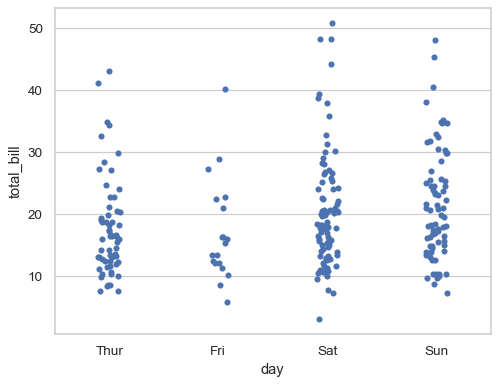Prior to version 0.12, the levels of the categorical variable had different colors by default. To get the same effect, assign the `hue` variable explicitly:

```sns.stripplot(data=tips, x="total_bill", y="day", hue="day", legend=False)
```Or you can assign a distinct variable to `hue` to show a multidimensional relationship:

```sns.stripplot(data=tips, x="total_bill", y="day", hue="sex")
```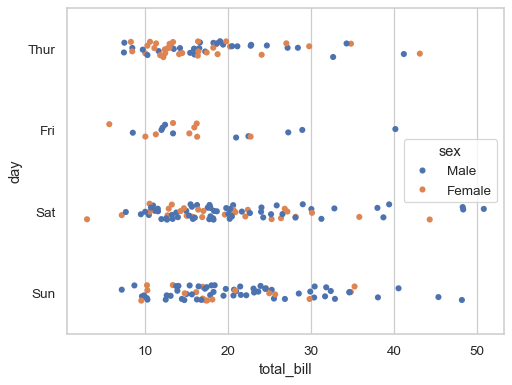If the `hue` variable is numeric, it will be mapped with a quantitative palette by default (note that this was not the case prior to version 0.12):

```sns.stripplot(data=tips, x="total_bill", y="day", hue="size")
```Use `palette` to control the color mapping, including forcing a categorical mapping by passing the name of a qualitative palette:

```sns.stripplot(data=tips, x="total_bill", y="day", hue="size", palette="deep")
```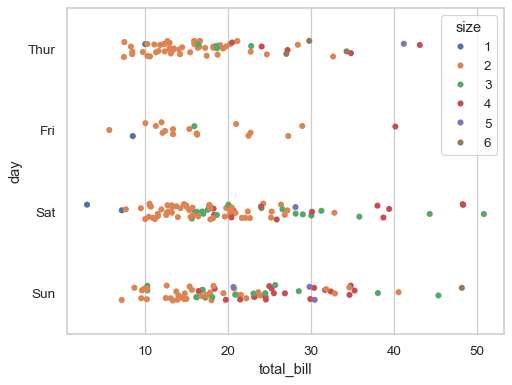By default, the different levels of the `hue` variable are intermingled in each strip, but setting `dodge=True` will split them:

```sns.stripplot(data=tips, x="total_bill", y="day", hue="sex", dodge=True)
```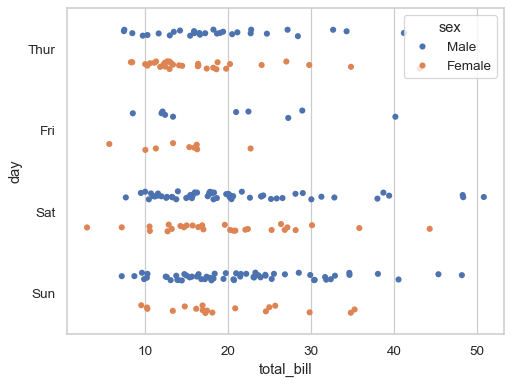The random jitter can be disabled by setting `jitter=False`:

```sns.stripplot(data=tips, x="total_bill", y="day", hue="sex", dodge=True, jitter=False)
```If plotting in wide-form mode, each numeric column of the dataframe will be mapped to both `x` and `hue`:

```sns.stripplot(data=tips)
```To change the orientation while in wide-form mode, pass `orient` explicitly:

```sns.stripplot(data=tips, orient="h")
```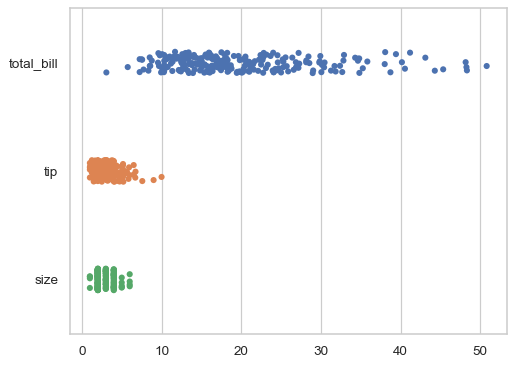The `orient` parameter is also useful when both axis variables are numeric, as it will resolve ambiguity about which dimension to group (and jitter) along:

```sns.stripplot(data=tips, x="total_bill", y="size", orient="h")
```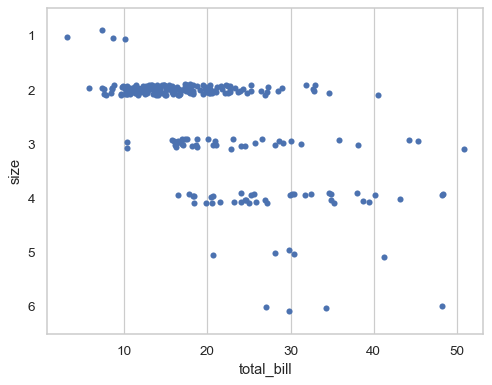By default, the categorical variable will be mapped to discrete indices with a fixed scale (0, 1, …), even when it is numeric:

```sns.stripplot(
data=tips.query("size in [2, 3, 5]"),
x="total_bill", y="size", orient="h",
)
```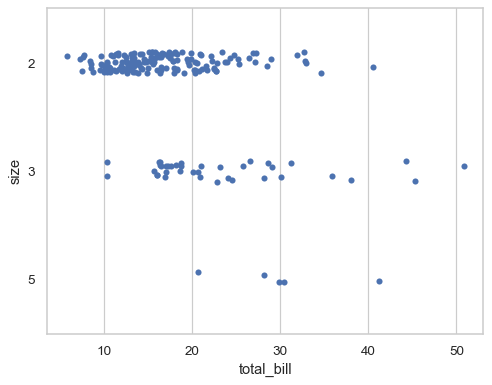To disable this behavior and use the original scale of the variable, set `native_scale=True`:

```sns.stripplot(
data=tips.query("size in [2, 3, 5]"),
x="total_bill", y="size", orient="h",
native_scale=True,
)
```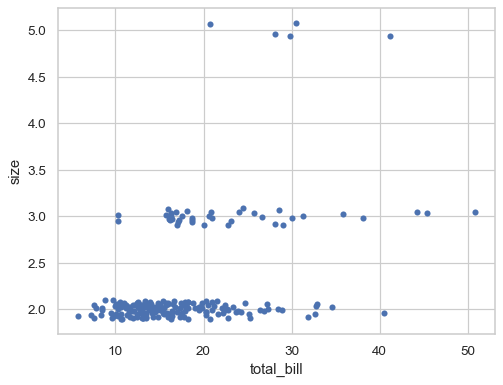Further visual customization can be achieved by passing keyword arguments for `matplotlib.axes.Axes.scatter()`:

```sns.stripplot(
data=tips, x="total_bill", y="day", hue="time",
jitter=False, s=20, marker="D", linewidth=1, alpha=.1,
)
```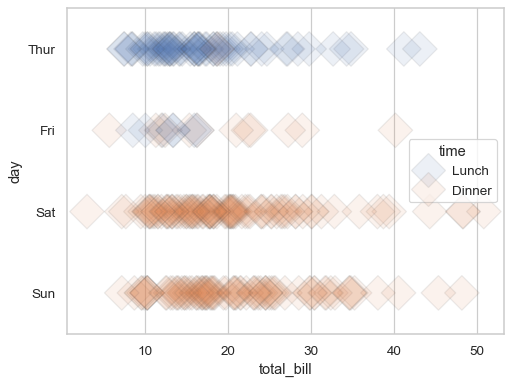To make a plot with multiple facets, it is safer to use `catplot()` than to work with `FacetGrid` directly, because `catplot()` will ensure that the categorical and hue variables are properly synchronized in each facet:

```sns.catplot(data=tips, x="time", y="total_bill", hue="sex", col="day", aspect=.5)
```